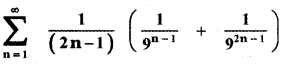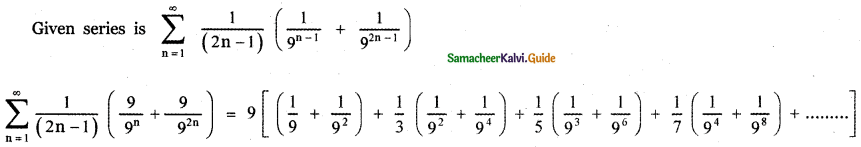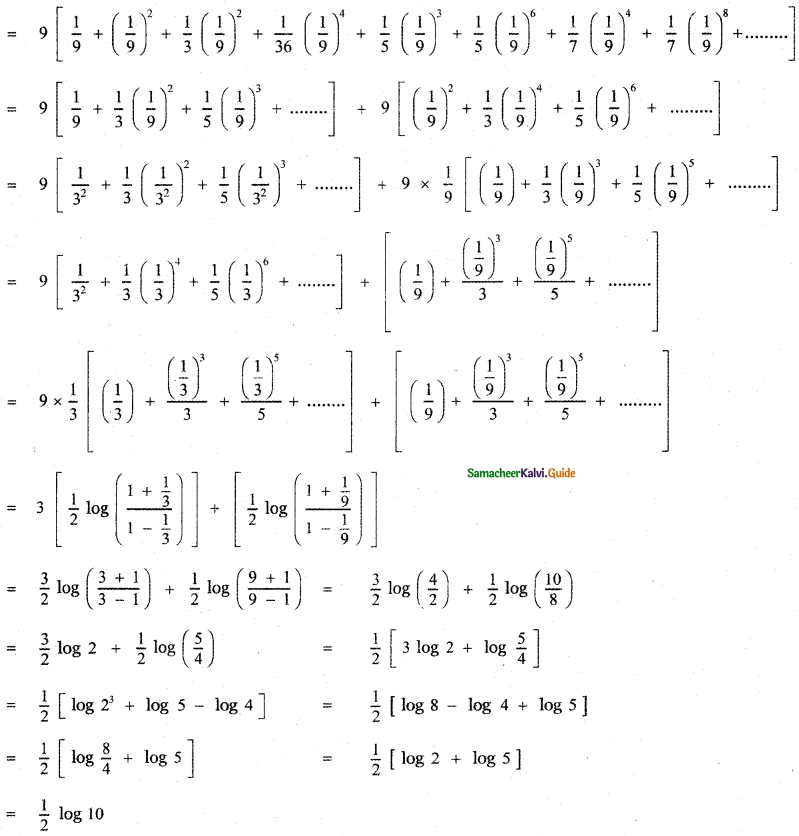Tamilnadu State Board New Syllabus Samacheer Kalvi 11th Maths Guide Pdf Chapter 5 Binomial Theorem, Sequences and Series Ex 5.4 Text Book Back Questions and Answers, Notes.

## Tamilnadu Samacheer Kalvi 11th Maths Solutions Chapter 5 Binomial Theorem, Sequences and Series Ex 5.4

Having trouble working out with the Binomial theorem? You’ve come to the right place, our Binomial Expansion Calculator is here to save the day for you.

Question 1.
Expand the following in ascending powers of x and find the condition on x for which the binomial expansion is valid.
(i) $$\frac{1}{5+x}$$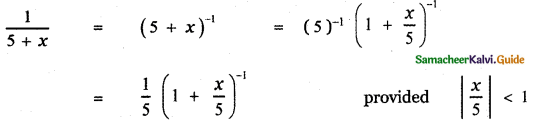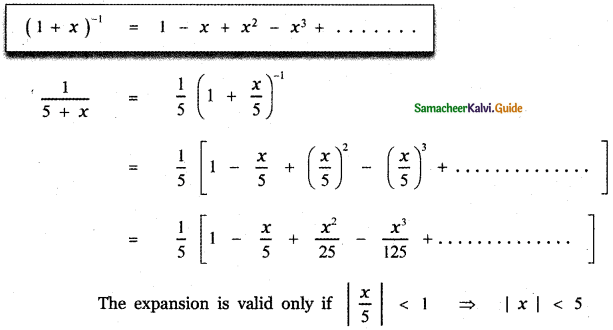(ii) $$\frac{2}{(3+4 x)^{2}}$$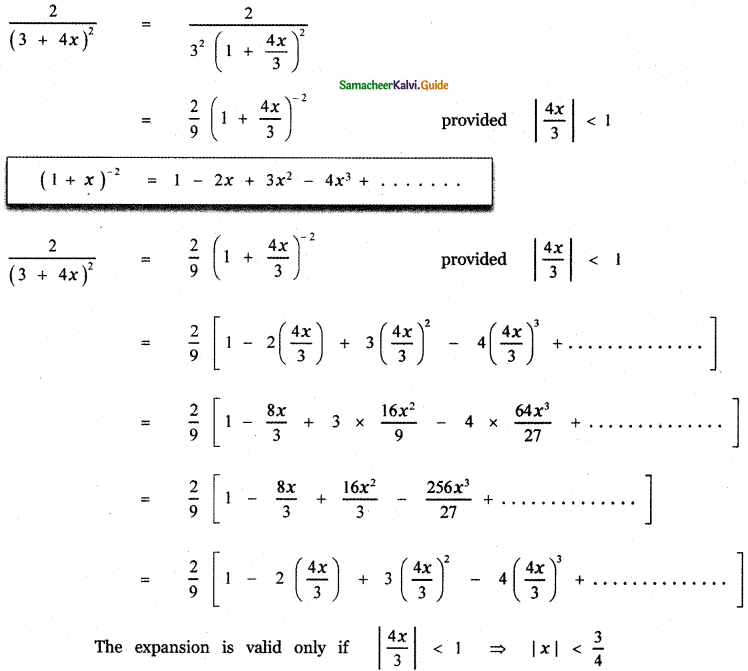(iii) (5 + x2)2/3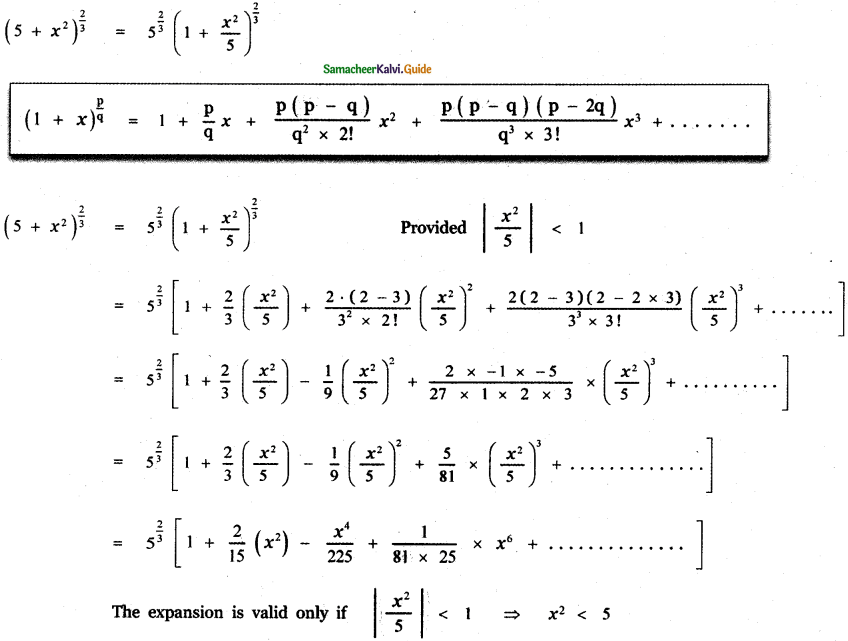(iv) $$(x+2)^{-\frac{2}{3}}$$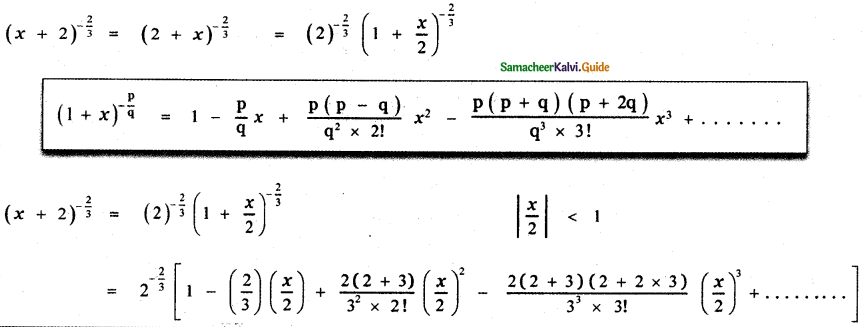Question 2.
Find $$\sqrt{1001}$$ approximately. (two decimal places)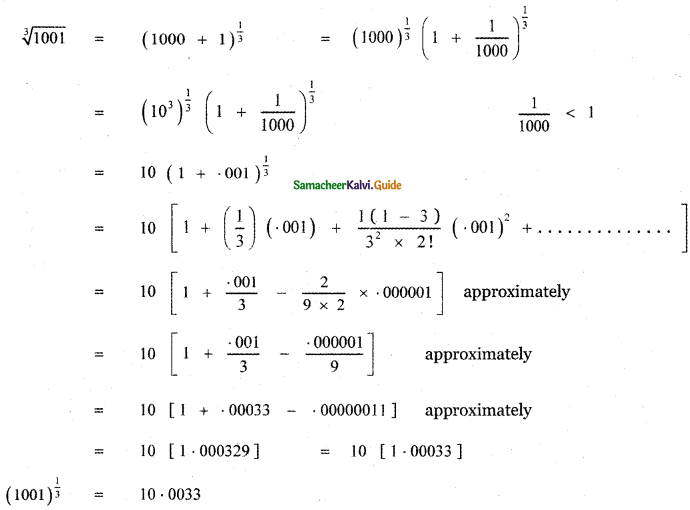Question 3.
Prove that $$\sqrt{x^{3}+6}-\sqrt{x^{3}+3}$$ is approximately equal to $$\frac{1}{x^{2}}$$ when x is sufficiently large.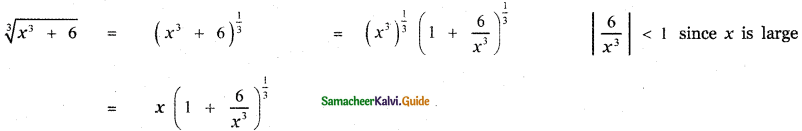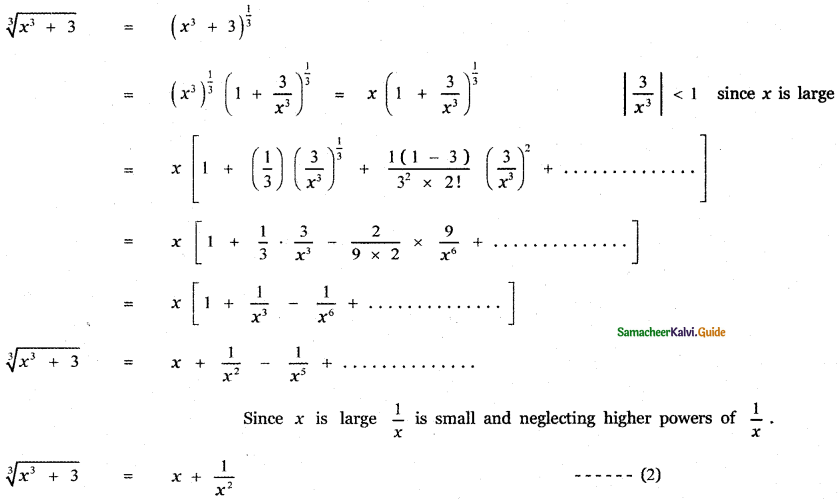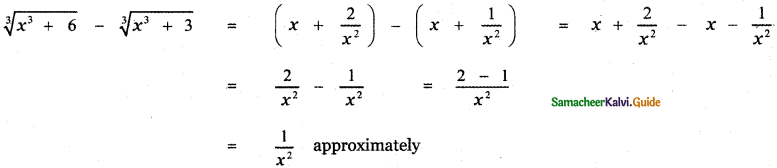Question 4.
Prove that $$\sqrt{\frac{1-x}{1+x}}$$ is approximately equal to 1 – x + $$\frac{x^{2}}{2}$$ when x is very small.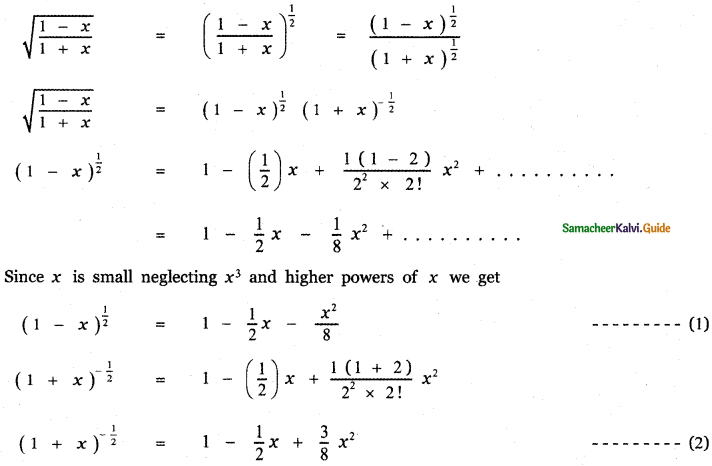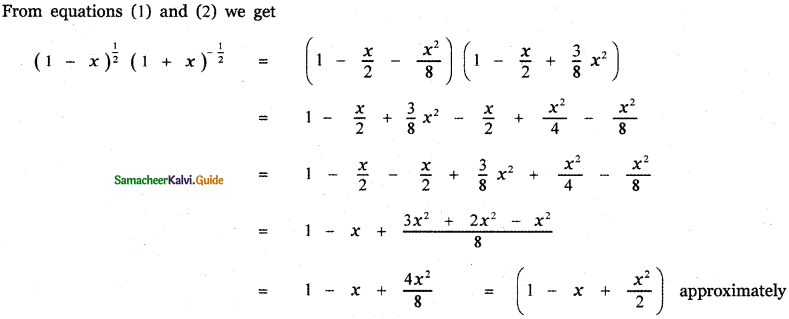Question 5.
Write the first 6 terms of the exponential series
(i) e5x(ii) e-2x(iii) ex/2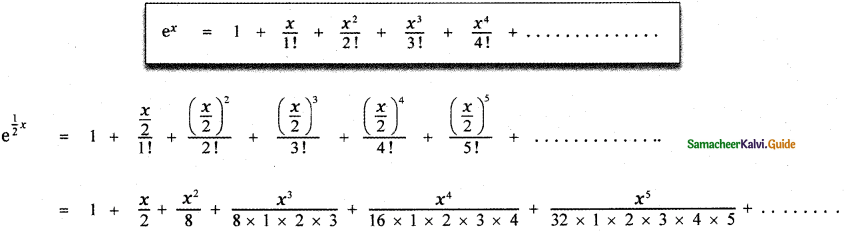Question 6.
Write the first 4 terms of the logarithmic series.
(i) log (1 + 4x)
(ii) log (1 – 2x)
(iii) log $$\left(\frac{1+3 x}{1-3 x}\right)$$
(iv) log $$\left(\frac{1-2 x}{1+2 x}\right)$$
Find the intervals on which the expansions are valid.
(i) log ( 1 + 4x )(ii) log (1 – 2x)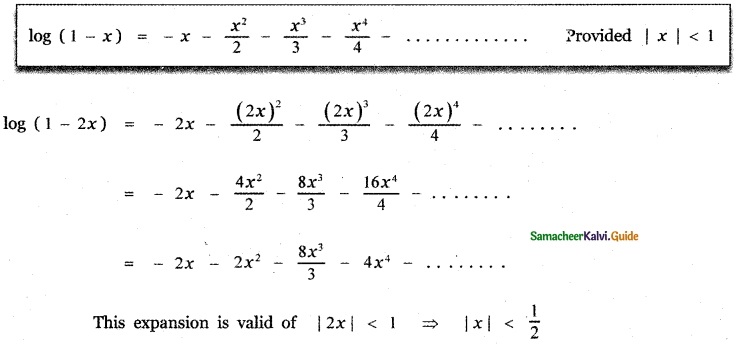(iii) log $$\left(\frac{1+3 x}{1-3 x}\right)$$(iv) log $$\left(\frac{1-2 x}{1+2 x}\right)$$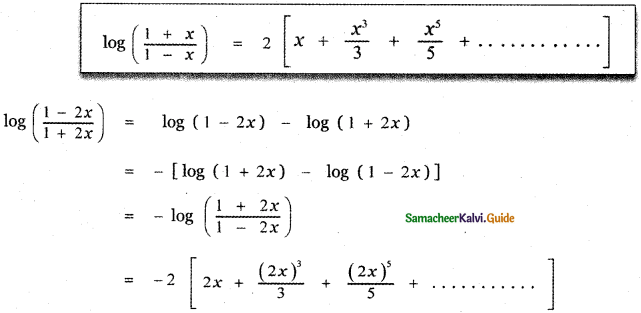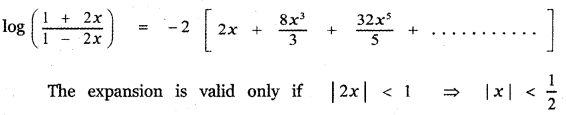Question 7.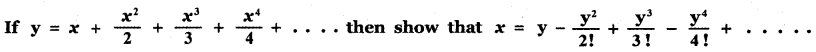Question 8.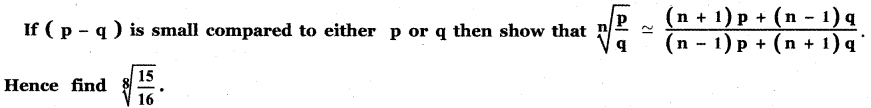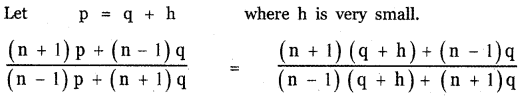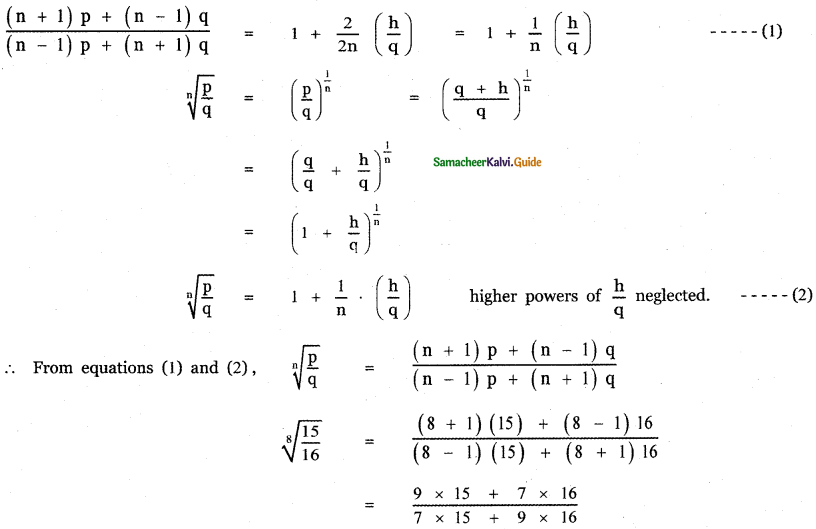Question 9.
Find the coefficient of x4 in the expansion of $$\frac{3-4 x+x^{2}}{e^{2 x}}$$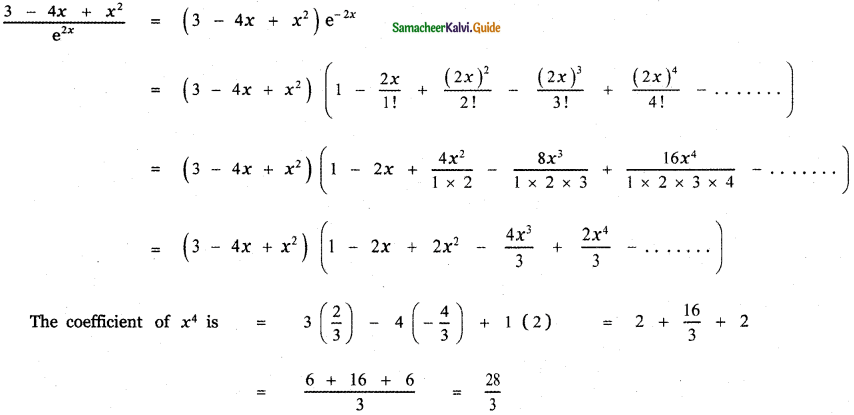Find the value of